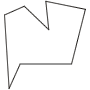index: click on a letter A B C D E F G H I J K L M N O P Q R S T U V W X Y Z A to Z index index: subject areas numbers & symbols sets, logic, proofs geometry algebra trigonometry advanced algebra & pre-calculus calculus advanced topics probability & statistics real world applications multimedia entrieswww.mathwords.com about mathwords website feedback

Polygon

A closed plane figure for which all sides are line segments. The name of a polygon describes the number of sides. A polygon which has all sides mutually congruent and all angles mutually congruent is called a regular polygon.

 Number of sides Polygon namepentagonregular undecagonoctagon 3 triangle 4 quadrilateral 5 pentagon 6 hexagon 7 heptagon 8 octagon 9 nonagon 10 decagon 11 undecagon 12 dodecagon n n-gon